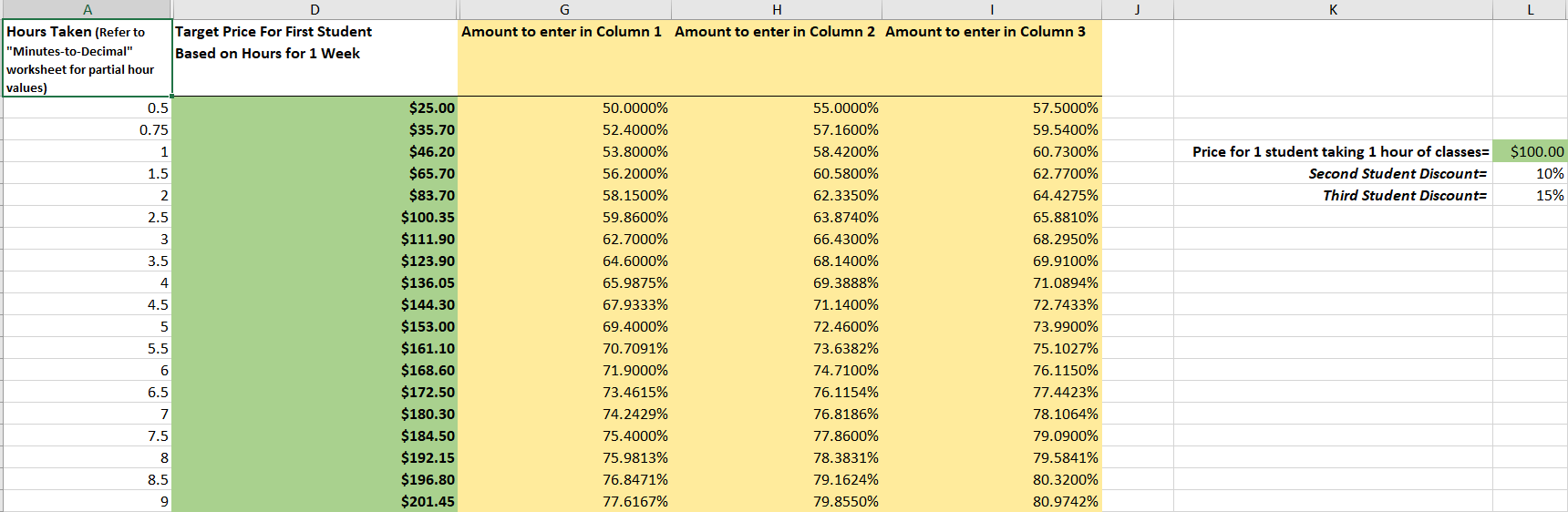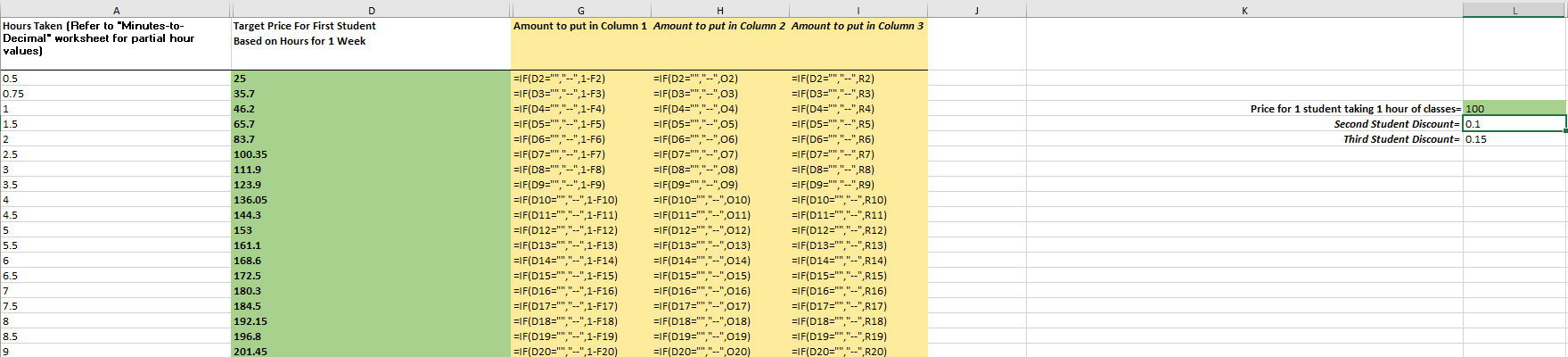# How Do I Set Up Hourly Billing in iClassPro?

Learn how to configure iClassPro to bill based on the number of hours for which a student or family is enrolled, including calculating your hourly rate and discounts.

#### What is hourly billing?

"Hourly billing" is when your tuition price is based on the total number of hours a student or family attends classes during a given week.  These hours are then totaled over the billing period and charged or discounted accordingly.

There are three basic ways to bill hourly in iClassPro:

• If you charge the same hourly rate regardless of how many hours are taken, you can simply select "Hourly" in answer to the question "How do you bill?" on the Class "Billing" tab and then attach a flat tuition amount to the class.
• Example: you always charge a flat amount of \$35 per hour.
• NOTE: If you offer a flat multi-hour/multi-sibling discount, you can also attach a Discount Schedule to the class.
• If you use a sliding scale to issue a discount based on the number of hours taken, you can select "Hourly" in answer to the question "How do you bill?" on the Class "Billing" tab and then attach a Tuition Schedule to the class (instead of a flat tuition).
• Example: you charge \$35 per hour for up to 2 hours of classes, then \$30 per hour after that.  You would create a Tuition Schedule reflecting these discounts and attach that to the class.
• In some special cases, it may be more desirable to use a flat tuition amount on the class, then use a Discount Schedule to arrive at the price you wish to charge.

#### Should I bill hourly in iClassPro?

The answer to this question is usually pretty simple. If you bill or discount customers by the number of hours an individual student or family is enrolled, you’re going to need to also bill hourly to get to the appropriate discounted amounts.

#### How do I figure out the hourly rate/base tuition?

For the best results, your hourly rate (or base tuition) should be equal to or more than the amount you charge for your shortest hourly class. (Because discounts cannot add to a tuition, it is always important to start with the most expensive classes - which are usually the shortest in length.)

For example, if the shortest class you offer is a \$40 half-hour class, your base hourly rate would need to be at least \$80.

Why? That way, if your hour long classes run \$60 each, I can create a discount for \$20 or 25% for a full hour class. If you used \$60 per hour instead, the half-hour class would have worked out to an un-discounted amount of \$30 (60/2 = 30) —which is \$10 short of what you actually wish to charge.

This amount will be used as the Tuition (SETTINGS>SETUP>CLASS SETTINGS>TUITIONS) which is attached to the class on the "Billing" tab. Additionally, each hourly class will need to be set up with the following options:

• How do you bill?
• Hourly
• How often will you bill for the class?
• Month/Session
• This class calculates prices by the number of hours in a...
• Week
• Do you allow discounts?
• Yes (if you are not using a Tuition Schedule for multi-hour/multi-sibling discounts)

Proration and tax settings can be determined on a class-by-class basis.

You will need to adjust your hourly rate if any of the discounts in Columns G-I of your spreadsheet below show a negative discounted percentage. This indicates that in order to come up with the tuition amount you entered, you would need to charge more than the current rate.

Great! Half the work is over. At this point, it’s easiest to use a program such as Microsoft Excel or other spreadsheet software to use formulas to calculate your discounts.

Screenshots based on this spreadsheet are displayed below. If you are using Firefox and these images appear blurry, try zooming in or out using CTRL + or CTRL - , respectively.

Calculations View:Formula View:Based on the previous example, this table illustrates the discounts at my gym. For detailed information about how this spreadsheet is set up, read the descriptions below.

• Column A: Lists the different totals of hours that students can take during a week (in decimal format).
• For additional help with entering decimal values for specific class lengths, see the "Minutes-to-Decimals Worksheet."
• Column D: The Target Price is how much you wish to charge Student 1 for the number of hours per week listed in Column A.
• Column G: The amount to enter in Column 1 of your Discount Schedule, represented as a Discounted Percentage of tuition. This is the discount that will be given to Student 1.
• Column H: The amount to enter in Column 2 of your Discount Schedule, represented as a Discounted Percentage of tuition. This is the discount that will be given to Student 2, or to all students if you count students by Total and there are two active students in the family.
• Column I: The amount to enter in Column 3 of your Discount Schedule, represented as a Discounted Percentage of tuition. This is the discount that will be given to Student 3, or to all students if you count students by Total and there are three active students in the family.
• Column L:
• Cell L4: The base tuition amount if a student takes one hour of classes during the week.  This should be at least double the amount you charge for your highest-priced 1/2 hour class.
• Cell L5: The discount you wish to give if the family has a second student (or two total students) enrolled. This value should be entered as a percentage.
• Cell L6: The discount you wish to give if the family has a third student (or three total students) enrolled. This value should be entered as a percentage.

#### Setting Up the Discount Schedule

When entering your discounts into the iClassPro Discount Schedule (SETTINGS>SETUP>CLASS SETTINGS>DISCOUNT SCHEDULES) section for classes as percentages, remember to include the percent sign (%). You can enter percent discounts up to the fourth decimal place, such as 8.3333%.